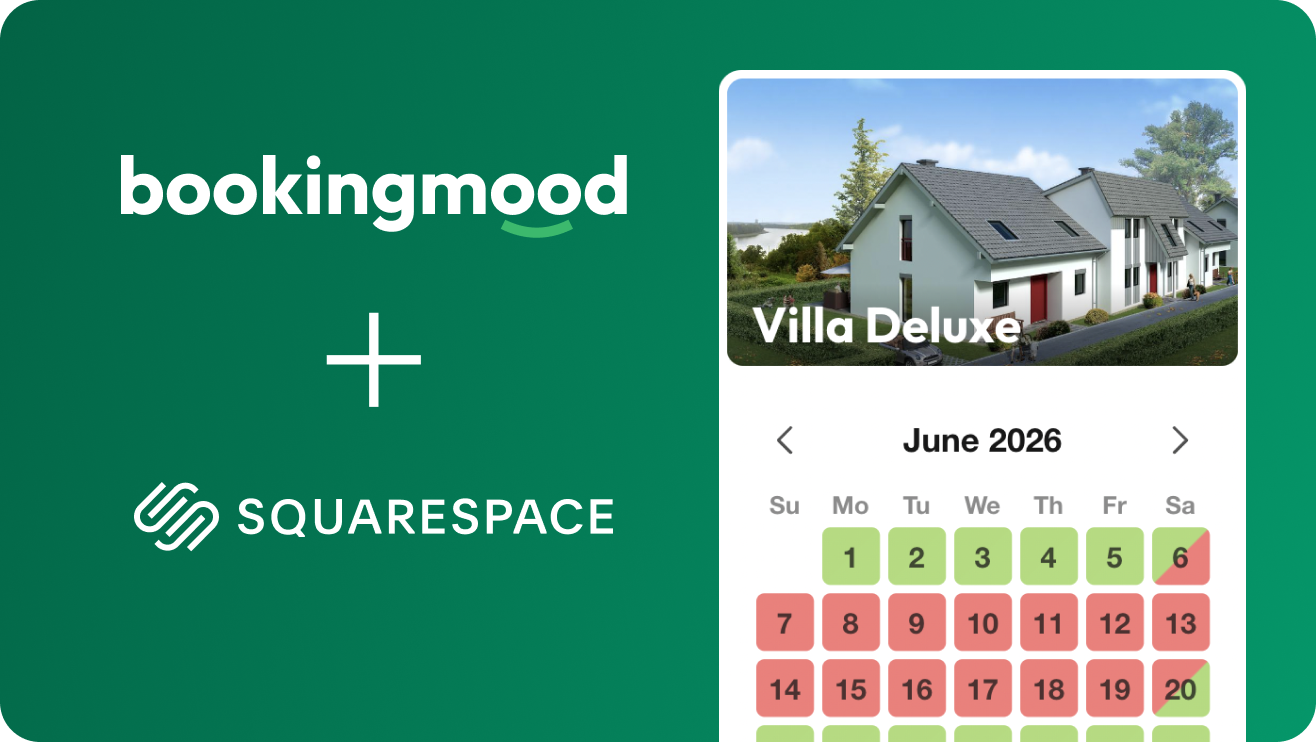# Booking calendar for Squarespace## Have you built your website with Squarespace, and are you looking for a booking calendar?self.__wrap_n=self.__wrap_n||(self.CSS&&CSS.supports("text-wrap","balance")?1:2);self.__wrap_b=(e,t,n)=>{let r=(n=n||document.querySelector(`[data-br="\${e}"]`)).parentElement,i=e=>n.style.maxWidth=e+"px";n.style.maxWidth="";let o=r.clientWidth,a=r.clientHeight,l=o/2-.25,s=o+.5,c;if(o){for(i(l),l=Math.max(n.scrollWidth,l);l+1<s;)i(c=Math.round((l+s)/2)),r.clientHeight===a?s=c:l=c;i(s*t+o*(1-t))}n.__wrap_o||"undefined"!=typeof ResizeObserver&&(n.__wrap_o=new ResizeObserver(()=>{self.__wrap_b(0,+n.dataset.brr,n)})).observe(r)};self.__wrap_n!=1&&self.__wrap_b(":R5aqpamlla:",1)

Bookingmood blends perfectly with your Squarespace website. No technical knowledge is required, Bookingmood works out of the box.

Embedding Bookingmood in Squarespace just takes 3 minutes!

View the manual

## When you embed Bookingmood your website:self.__wrap_n=self.__wrap_n||(self.CSS&&CSS.supports("text-wrap","balance")?1:2);self.__wrap_b=(e,t,n)=>{let r=(n=n||document.querySelector(`[data-br="\${e}"]`)).parentElement,i=e=>n.style.maxWidth=e+"px";n.style.maxWidth="";let o=r.clientWidth,a=r.clientHeight,l=o/2-.25,s=o+.5,c;if(o){for(i(l),l=Math.max(n.scrollWidth,l);l+1<s;)i(c=Math.round((l+s)/2)),r.clientHeight===a?s=c:l=c;i(s*t+o*(1-t))}n.__wrap_o||"undefined"!=typeof ResizeObserver&&(n.__wrap_o=new ResizeObserver(()=>{self.__wrap_b(0,+n.dataset.brr,n)})).observe(r)};self.__wrap_n!=1&&self.__wrap_b(":Rdaqpamlla:",1)

• Your visitors can make a booking inquiry directly via your Squarespace website
• Your Squarespace website site turns into a booking channel

## Bookingmood is 100% compatible with Squarespaceself.__wrap_n=self.__wrap_n||(self.CSS&&CSS.supports("text-wrap","balance")?1:2);self.__wrap_b=(e,t,n)=>{let r=(n=n||document.querySelector(`[data-br="\${e}"]`)).parentElement,i=e=>n.style.maxWidth=e+"px";n.style.maxWidth="";let o=r.clientWidth,a=r.clientHeight,l=o/2-.25,s=o+.5,c;if(o){for(i(l),l=Math.max(n.scrollWidth,l);l+1<s;)i(c=Math.round((l+s)/2)),r.clientHeight===a?s=c:l=c;i(s*t+o*(1-t))}n.__wrap_o||"undefined"!=typeof ResizeObserver&&(n.__wrap_o=new ResizeObserver(()=>{self.__wrap_b(0,+n.dataset.brr,n)})).observe(r)};self.__wrap_n!=1&&self.__wrap_b(":Rhaqpamlla:",1)

We've tested Bookingmood with Squarespace and it works great. You only have to embed your Bookingmood widget once. The rest is managed from the Bookingmood dashboard. From there you can edit the availability and manage the bookings. Updates to the booking calendar are automatically applied and will be visible on your Squarespace site just after you hit Save!

View all features